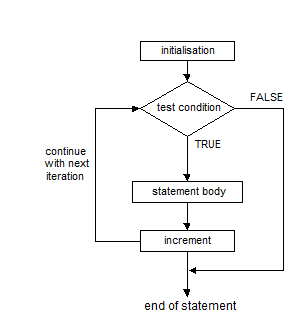## For Loop In C Language With Examples

### for statement :

The for statement is most often used in situations where the programmer knows in advance how many times a particular set of statements are to be repeated. The for statement is sometimes termed a counted loop.

Syntax
```for ( [initialisation] ; [condition] ; [increment] )
[statement body] ;```

initialisation :- this is usually an assignment to set a loop counter variable for example.

condition :- determines when loop will terminate.

increment :- defines how the loop control variable will change each time the loop is executed.

statement body :- can be a single statement, no statement or a block of statements.

The for statement executes as follows :-NOTE : The square braces above are to denote optional sections in the syntax but are not part of the syntax. The semi-colons must be present in the syntax.

For Example : Program to print out all numbers from 1 to 100.

CODE/PROGRAM/EXAMPLE
```#include <stdio.h>
void main()
{
int x ;

for ( x = 1;  x <= 100; x++ )
printf( "%d\n", x ) ;
}```

Curly braces are used in C to denote code blocks whether in a function as in main() or as the body of a loop.

For Example :- To print out all numbers from 1 to 100 and calculate their sum.

CODE/PROGRAM/EXAMPLE
```#include <stdio.h>
void main()
{
int x, sum = 0 ;

for ( x = 1; x <= 100; x++ )
{
printf( "%d\n", x ) ;
sum += x ;
}
printf( “\n\nSum is %d\n”, sum ) ;
}```

Multiple Initialisations:

C has a special operator called the comma operator which allows separate expressions to be tied together into one statement.

For example it may be tidier to initialise two variables in a for loop as follows :-

Syntax
```for ( x = 0, sum = 0; x <= 100; x++ )
{
printf( "%d\n", x) ;
sum += x ;
}```

Any of the four sections associated with a for loop may be omitted but the semi-colons must be present always.

Syntax
```For Example :-
for ( x = 0; x < 10;   )
printf( "%d\n", x++ ) ;
...
x = 0 ;
for (  ; x < 10; x++ )
printf( "%d\n", x ) ;```

An infinite loop may be created as follows

Syntax
```for ( ;  ;  )
statement body ;```

or indeed by having a faulty terminating condition.

Sometimes a for statement may not even have a body to execute as in the following example where we just want to create a time delay.

Syntax
```for ( t = 0; t < big_num ; t++ )  ;
or we could rewrite the example given above as follows
for ( x = 1; x <= 100; printf( "%d\n", x++ ) ) ;```

The initialisation, condition and increment sections of the for statement can contain any valid C expressions.

Syntax
```for ( x = 12 * 4 ; x < 34 / 2 * 47 ; x += 10 )
printf( “%d “, x ) ;```

It is possible to build a nested structure of for loops, for example the following creates a large time delay using just integer variables.

Syntax
```unsigned int x, y ;
for ( x = 0; x < 65535; x++ )
for ( y = 0; y < 65535; y++ ) ;

1    2    3    4    5
2    3    4    5    6
3    4    5    6    7
4    5    6    7    8
5    6    7    8    9```

For Example : Program to produce the following table of values

CODE/PROGRAM/EXAMPLE
```#include <stdio.h>
void main()
{
int j, k ;

for ( j = 1; j <= 5; j++ )
{
for ( k = j ; k < j + 5; k++ )
{
printf( "%d  ", k ) ;
}
printf( "\n" ) ;
}
}```
#for_loop_in_c_language #for_loop_in_c #for_loop_c_programming #for_loop_in_c_programming_example #for_loop_syntax_in_c #for_loop_example_in_c #for_loop_flowchart_in_c #Multiple_initialisations_in_for_loop

### (New page will open, for Comment)

Not yet commented...